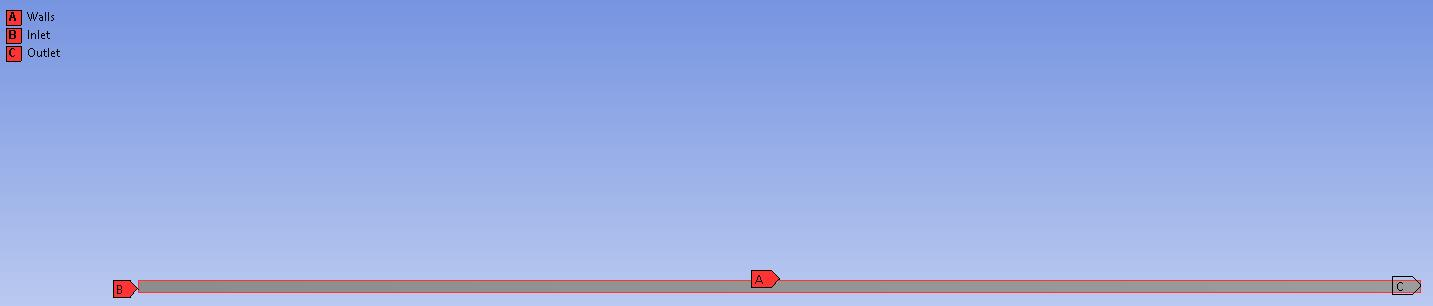# Incorrect wall temperature in channel flow with constant heat flux BCs

Hi all,

I am trying to reproduce my experimental results for air flow through a channel with constant heat flux on sidewalls. My experimental setup is as following:

Fully developed air flow with velocity of 2.6 (m/s) goes through a channel with 5 mm width, 152 mm height and 0.5 m length. The air flow is laminar. There are heaters on sidewalls that provide a uniform 50 W heat to air flow. Top and bottom walls are insulated. The experiment conserves both mass and energy. There are six thermocouples in inlet and outlet for measuring air temperature. Also, six thermocouples are used for measuring wall's temperature in inlet and outlet.

For simulation, I used SpaceCIaim and made a two-dimensional channel with 0.5 m length and 5 mm width. Then, in Ansys Meshing, I made a constructed mesh consists of 25,000 nodes and named lines for boundaries (Following image shows the boundaries). The mesh was converted to Fluent.In Fluent, I chose steady-state and pressure-based solver. For boundary conditions, I chose velocity-inlet and outflow. To get a fully-developed inlet flow, I ran simulation for momentum equations. After convergence, I inserted the outlet velocity to inlet. Then, the energy equation was turned on. For thermal boundary conditions, temperature inlet of T=21.95 C and a constant heat flux of 326 W/m^2 were used. The Solution setting was not changed and a hybrid initialization method was used.

After convergence, I compared results with my experiments. Experimental result shows inlet wall temperature of 40 C and my simulation result is 30 C. Also, outlet wall temperature in experiment is 51.8 C and in Fluent is 56.7 C.

My objective is to compare Nusselt number between experimental and numerical results, and validate numerical results. However, the difference between walls' temperature in experimental and numerical results are significant. I was wondering if someone could give me some ideas on where I might be wrong in Fluent simulations?

Any help would be appreciated.

Thank you.

• edited February 2020

Check mesh resolution and that you have the velocity profile you're expecting.

Also check heat input in the two cases. In 2d Fluent assumes the domain is 1m thick so have you got the same amount of air mass flow, velocity & energy as the experiment?

• edited February 2020

Thank you rwoolhou for your response.

I refined mesh up to 100,000 and the result was same. I am using a fully-developed velocity profile at inlet and the difference between inlet and outlet velocity profile is less than %1.

I have used similar inputs to experiments for my simulations.

I applied your idea about heat flux at the walls. I divided the wall into three sections and applied 720 (W/m^2) for first part (0.15 m long), 210 (W/m^2) for second part (0.15 m long), 120 (W/m^2) for third part (0.2 m long). It seems that the results are improved, but there is still a %20 difference between experimental and CFD results.

Could you let me know where else I should be looking for source of error in my simulation?